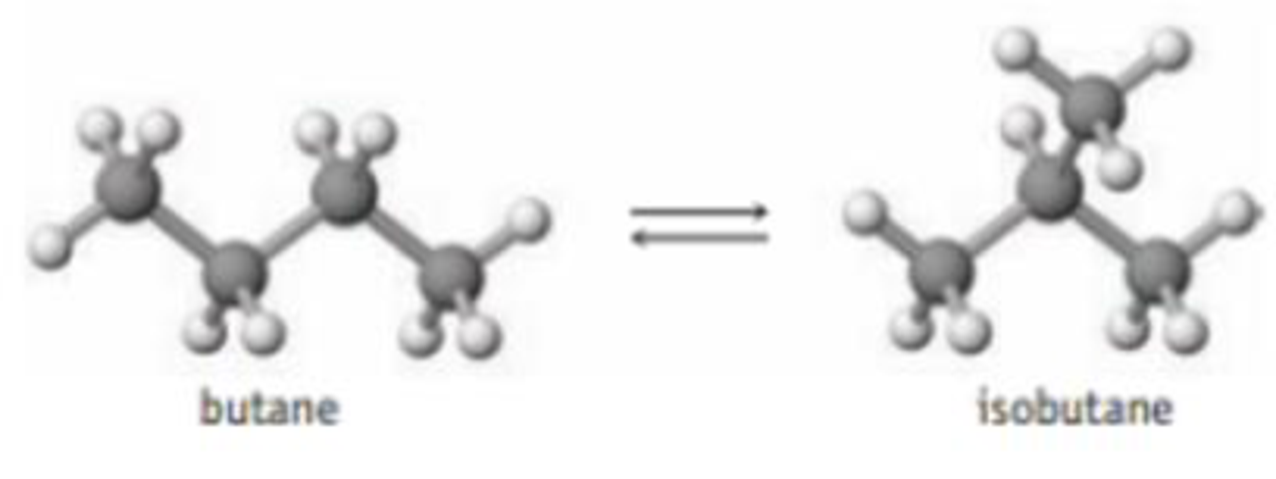Chapter 15, Problem 13PS

Chapter
Section
Textbook Problem

The value of Kc for the interconversion of butane and isobutane is 2.5 at 25 °C.If you place 0.017 mol of butane in a 0.50-L flask at 25 °C and allow equilibrium to be established, what will be the equilibrium concentrations of the two forms of butane?

Interpretation Introduction

Interpretation: The equilibrium concentration of two form of butane when the system comes to equilibrium has to be determined.

Concept introduction:

• Equilibrium constant: At equilibrium the ratio of products to reactants has a constant value. And it is represented by the letter K.

For a general reaction, aA+bBcC+dD

The equilibrium constant Kc=[C]c[D]d[A]a[B]b, where a, b, c and d are the stoichiometric coefficients of reactant and product in the reaction. Concentration value for solid substance is 1.

If the value of Kc and the concentration of any of the reactant of a reaction is known then the concentration of product can be determined by multiplying Kc with the concentration of reactant.

• Concentration =AmountofsubstanceVolume
• ICE (reaction initial concentration equilibrium) table is mainly used to calculate the value of K for a reaction. This table contains the concentration of reactant and product in various stage of reaction.
Explanation

The given equilibrium set up contains butane and isobutene. And the value of Kc for the interconversion of butane and isobutane is 2.5at250C

The concentration of butane = 0.017mol0.5L=0.034mol/L

By using these concentration ICE table for this reaction can be constructed as follows,

 Reaction                      Butane              ⇄          Isobutane Initial concentration(mol/L) 0

Still sussing out bartleby?

Check out a sample textbook solution.

See a sample solution

The Solution to Your Study Problems

Bartleby provides explanations to thousands of textbook problems written by our experts, many with advanced degrees!

Get Started

Find more solutions based on key concepts